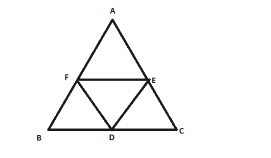# The line segments joining the midpoints of the sides of a triangle form four triangles, each of which isQuestion:

The line segments joining the midpoints of the sides of a triangle form four triangles, each of which is
(a) congruent to the original triangle
(b) similar to the original triangle
(c) an isosceles triangle
(d) an equilateral triangle

Solution:

(b) similar to the original triangle

The line segments joining the midpoints of the sides of a triangle form four triangles, each of which is similar to the original triangle.